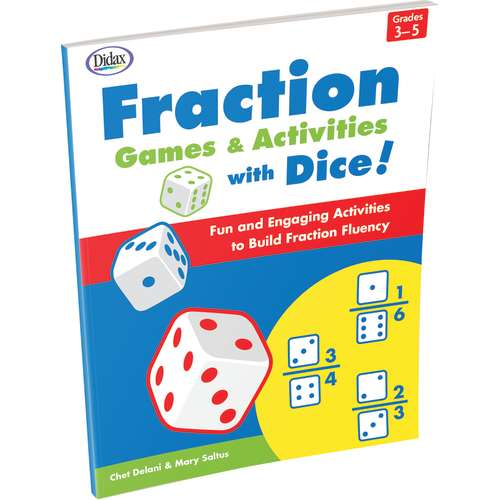•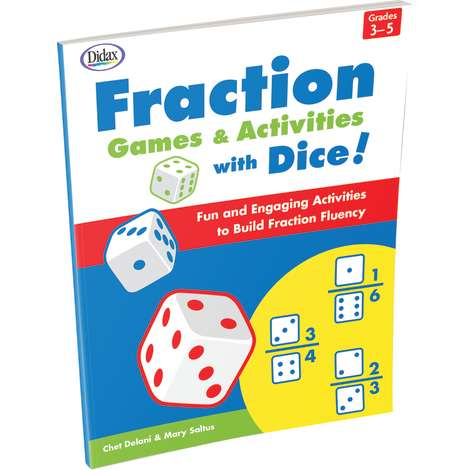•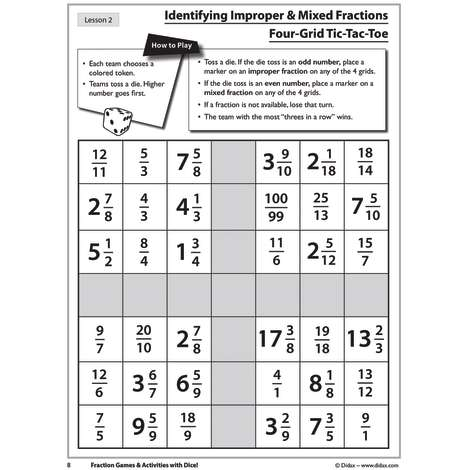•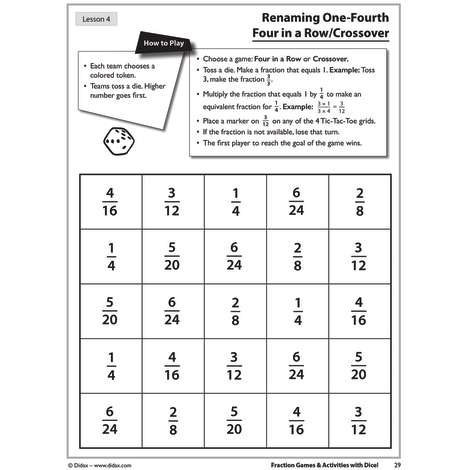•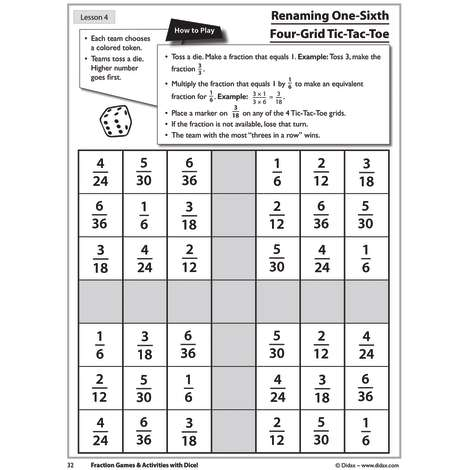Fraction Games & Activities with Dice

Product Number: DD-211187
\$16.99
The more than 70 dice activities in this book go beyond conventional algorithms to awaken students’ commonsense grasp of frac...
Quantity

The more than 70 dice activities in this book go beyond conventional algorithms to awaken students’ commonsense grasp of fractions. Students use fraction bars, number lines and competitive games (Tic-Tac-Toe, Four in a Row, Cross Over) to add, subtract, multiply and divide fractions; recognize proper, improper and mixed fractions; and work with equivalent fractions and inequalities.

Each section includes detailed teacher notes for teaching fractions for understanding.

120 pages.
DD-211187